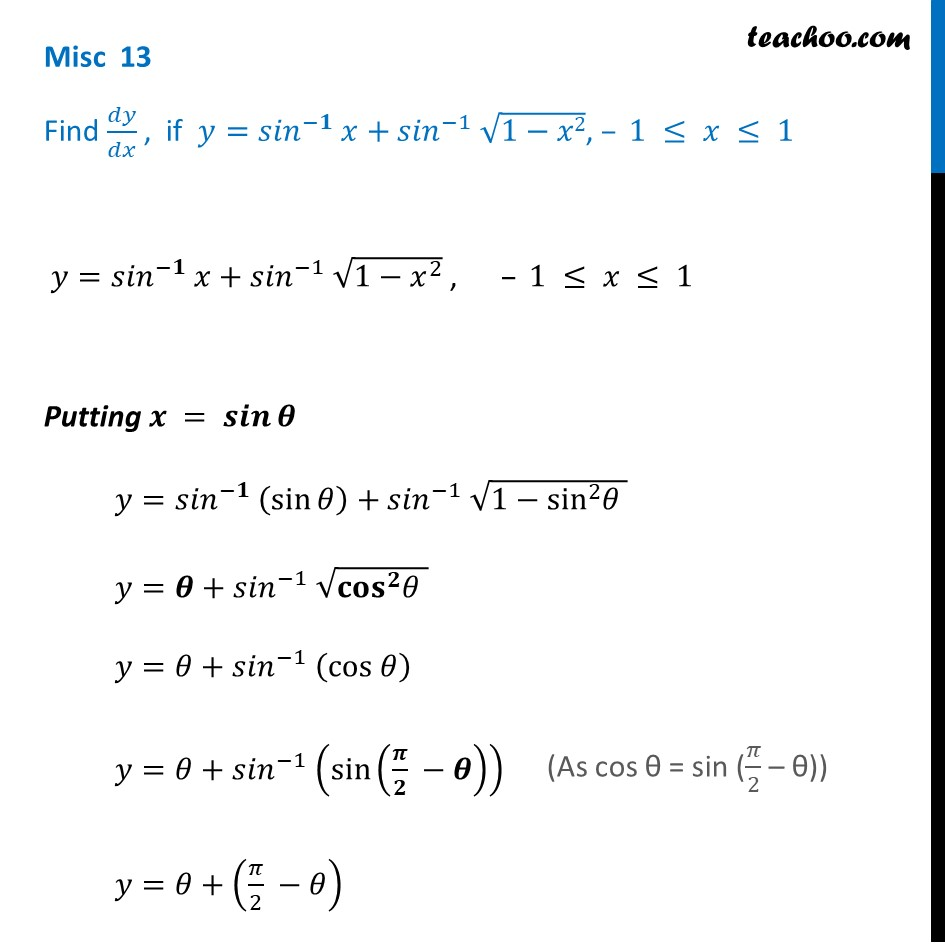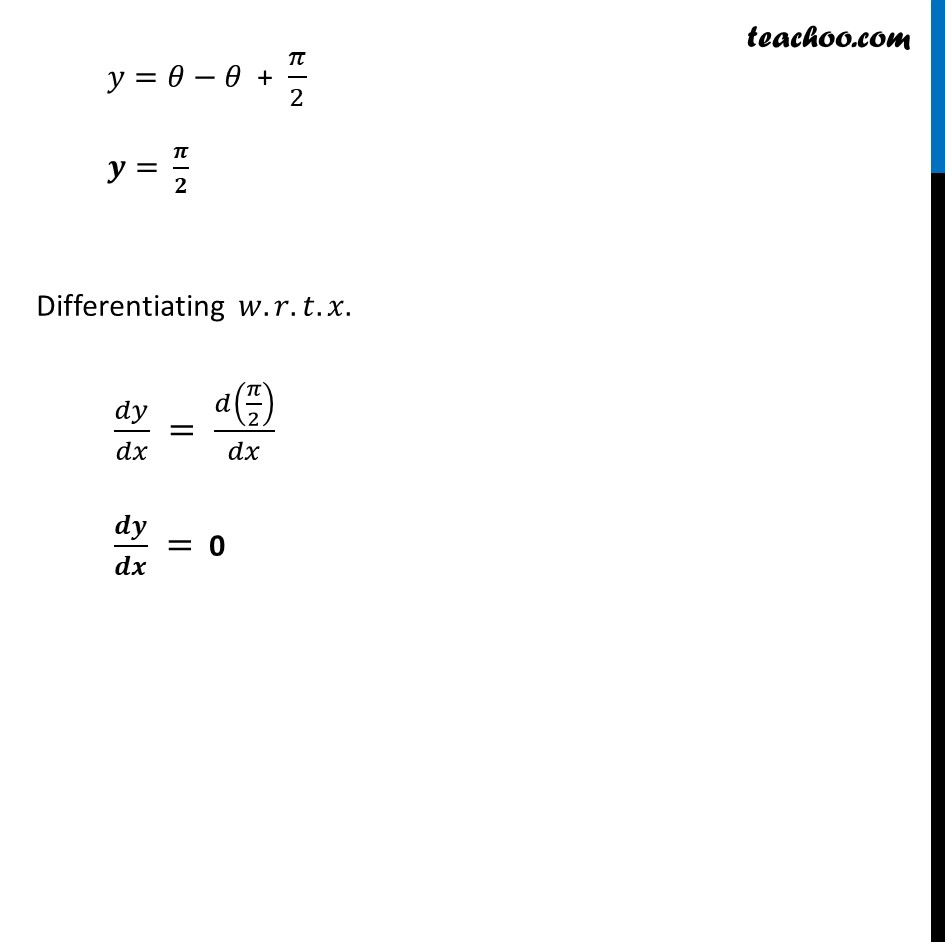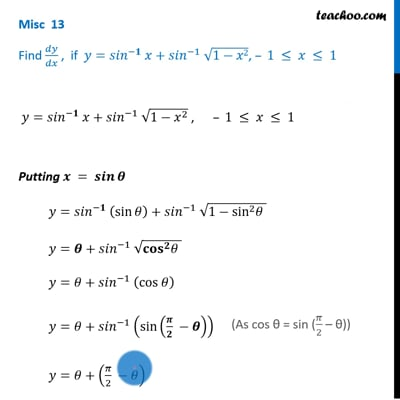Miscellaneous

Chapter 5 Class 12 Continuity and Differentiability
Serial order wiseThis video is only available for Teachoo black users

Solve all your doubts with Teachoo Black (new monthly pack available now!)

### Transcript

Misc 13 Find 𝑑𝑦/𝑑𝑥 , if 𝑦=〖𝑠𝑖𝑛〗^(−𝟏) 𝑥+〖𝑠𝑖𝑛〗^(−1) √(1−𝑥2), – 1 ≤ 𝑥 ≤ 1 𝑦=〖𝑠𝑖𝑛〗^(−𝟏) 𝑥+〖𝑠𝑖𝑛〗^(−1) √(1−𝑥^2 ) , – 1 ≤ 𝑥 ≤ 1 Putting 𝒙 = 𝒔𝒊𝒏⁡𝜽 𝑦=〖𝑠𝑖𝑛〗^(−𝟏) (sin⁡𝜃)+〖𝑠𝑖𝑛〗^(−1) √(1−sin^2 𝜃 ) 𝑦=𝜽+〖𝑠𝑖𝑛〗^(−1) √(〖𝐜𝐨𝐬〗^𝟐 𝜃 ) 𝑦=𝜃+〖𝑠𝑖𝑛〗^(−1) (cos 𝜃) 𝑦=𝜃+〖𝑠𝑖𝑛〗^(−1) (sin⁡(𝝅/𝟐 −𝜽) ) 𝑦=𝜃+ (𝜋/2 −𝜃) 𝑦=𝜃−𝜃 + 𝜋/2 𝒚= 𝝅/𝟐 Differentiating 𝑤.𝑟.𝑡.𝑥. 𝑑𝑦/𝑑𝑥 = 𝑑(𝜋/2)/𝑑𝑥 𝒅𝒚/𝒅𝒙 = 0